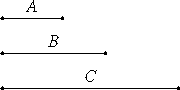# Proposition 6

If a number multiplied by itself makes a cubic number, then it itself is also cubic.

Let the number A multiplied by itself make the cubic number B.

I say that A is also cubic.

Multiply A by B to make C. Since, then, A multiplied by itself makes B, and multiplied by B makes C, therefore C is a cube. And, since A multiplied by itself makes B, therefore A measures B according to the units in itself.VII.Def.20

But the unit also measures A according to the units in it. Therefore the unit is to A as A is to B. And, since A multiplied by B makes C, therefore B measures C according to the units in A.

But the unit also measures A according to the units in it.

VII.Def.20

Therefore the unit is to A as B is to C. But the unit is to A as A is to B, therefore A is to B as B is to C.

And, since B and C are cubes, therefore they are similar solid numbers.

Therefore there are two mean proportional numbers between B and C. And B is to C as A is to B. Therefore there are two mean proportional numbers between A and B also.

VIII.23

And B is a cube, therefore A is also a cube.

Therefore, if a number multiplied by itself makes a cubic number, then it itself is also cubic.

Q.E.D.

## Guide

#### Outline of the proof

Assume that a2 is a cube. Since a3 is also a cube, therefore there are two mean proportionals between them (VIII.19). But we have the proportion a : a2 = a2:a3, so there are also two mean proportionals between a and a2 (VIII.8). And since a2 is a cube, therefore a is also a cube (VIII.23).

#### Use of this proposition

This proposition is used in IX.10.